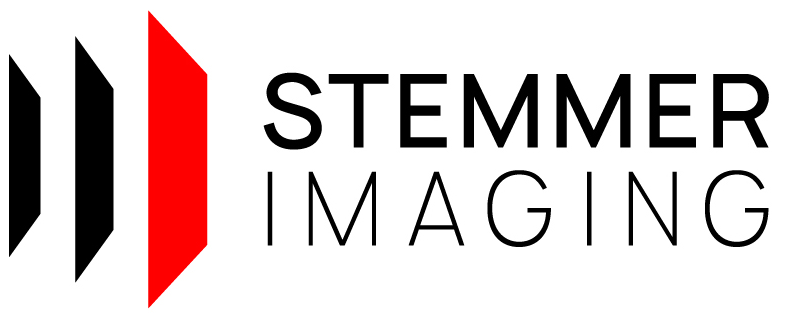CVB++ 14.0
Circle Class Referencefinal

Class representing a circle. More...

`#include <cvb/circle.hpp>`

## Public Member Functions

Circle (Point2D< double > center, double radius) noexcept
Create a circle. More...

Point2D< double > Center () const noexcept
Gets the center of the circle. More...

void SetCenter (Point2D< double > center) noexcept
Sets the center of the circle. More...

Gets the radius of the circle. More...

Sets the radius of the circle. More...

bool Contains (Point2D< double > point) const noexcept
Check whether a point is inside or outside a circle. More...

bool IsOnRadius (Point2D< double > point, double epsilon) const noexcept
Check whether a point is on the radius of a circle. More...

bool operator== (const Circle &circle) const noexcept
Compares to an other circle. More...

bool operator!= (const Circle &circle) const noexcept
Compares to an other circle. More...

## Detailed Description

Class representing a circle.

For the purpose of regression and other calculations.

## ◆ Circle()

 Circle ( Point2D< double > center, double radius )
inlinenoexcept

Create a circle.

Parameters
 [in] center Center of the circle. [in] radius Radius of the circle.
Exceptions
 Does not throw any exception.

## ◆ Center()

 Point2D< double > Center ( ) const
inlinenoexcept

Gets the center of the circle.

Returns
The center.
Exceptions
 Does not throw any exception.

## ◆ Contains()

 bool Contains ( Point2D< double > point ) const
inlinenoexcept

Check whether a point is inside or outside a circle.

Parameters
 [in] point Point to be checked.
Returns
bool True if the point is inside the circle, false otherwise.
Exceptions
 Does not throw any exception.

 bool IsOnRadius ( Point2D< double > point, double epsilon ) const
inlinenoexcept

Check whether a point is on the radius of a circle.

Parameters
 [in] point Point to be checked. [in] epsilon Maximum allowable deviation.
Returns
True if the point lies within the allowable deviation of the circle's radius, false otherwise.
Exceptions
 Does not throw any exception.

## ◆ operator!=()

 bool operator!= ( const Circle & circle ) const
inlinenoexcept

Compares to an other circle.

Parameters
 [in] circle Other circle.
Returns
True if not equal, otherwise false.
Exceptions
 Does not throw any exception.

## ◆ operator==()

 bool operator== ( const Circle & circle ) const
inlinenoexcept

Compares to an other circle.

Parameters
 [in] circle Other circle.
Returns
True if equal, otherwise false.
Exceptions
 Does not throw any exception.

inlinenoexcept

Gets the radius of the circle.

Returns
Exceptions
 Does not throw any exception.

## ◆ SetCenter()

 void SetCenter ( Point2D< double > center )
inlinenoexcept

Sets the center of the circle.

Parameters
 [in] center The new center.
Exceptions
 Does not throw any exception.